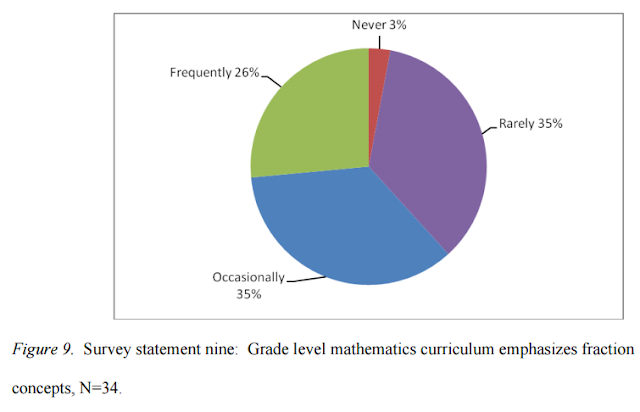### Adding Fractions and the Spiral Curriculum

Addition, subtraction, multiplication and division of fractions are inherently difficult. Hugues Lortie-Forgues, Jing Tian, and Robert S. Siegler explore the challenges of fractions in their recent review article, Why is learning fraction and decimal arithmetic so difficult?, in the journal Developmental Review. In a doctoral dissertation presented to the Educational Leadership Program of the College of Saint Elizabeth, Elissa Mains Scillieri seeks the answers to challenges in elementary school mathematics education from the teachers themselves. Among the findings from this survey by Scillieri is that grade school mathematics spirals thereby making it difficult for both students and teachers to focus and gain a deeper understanding.

The survey covers a school district in New Jersey. Scillieri draws her conclusion from the data she obtained from teachers about the curriculum. Scillieri presents these findings in the following graphs. The first one answers the question of whether fraction concepts are emphasized in elementary education.Above copied from Elissa Mains Scillieri, Elementary mathematics: Not so elementary
Seeing that dealing with fractions is well known to be problematic, it is surprising that only one out of four teachers says that this topic is emphasized. The second graph relates the results when the question is phrased in general terms. If fractions are not emphasized, this question basically inquires whether other topics have been covered in greater depth.Above copied from Elissa Mains Scillieri, Elementary mathematics: Not so elementary
Obviously, based on these results, classes hop from one topic to the next without requiring mastery or deeper understanding.

Another finding that is quite striking is presented in the graph shown below.Above copied from Elissa Mains Scillieri, Elementary mathematics: Not so elementary
A clear majority of teachers feel that the elementary mathematics curriculum covers a wide array of topics. The spiral curriculum tends to jump from one topic to another.

To appreciate this in specific terms, one may browse, for example, at the mathematics curriculum of Philippines' DepEd K to 12. This curriculum is an example of a spiral approach in teaching that jumps from topic to topic in the course of every year. For instance, if one simply keeps tab of when fractions are taught, one finds a coverage of fractions in first grade. After learning how to count and develop some rudimentary number sense, first grade pupils are introduced to the fractions 1/2 and 1/4. After this brief excursion, the students then proceed to study shapes, patterns, measurements and simple pictographs. Students encounter fractions again in second grade. This time, fractions are presented as dividing a whole into parts. Then the students work on straight and curved lines, symmetry, more patterns, more measurement and more pictographs. In third grade, students learn about odd and even numbers then they go into fractions that are either less than or greater than one. Equivalent fractions are also covered. Then, students receive more instruction on symmetry, patterns, measurement conversion, and bar graphs. It is in fourth grade that students learn how to add and subtract fractions.

As enumerated by Hugues Lortie-Forgues, Jing Tian, and Robert S. Siegler in their recent paper, dealing with fractions has inherent difficulties. On top of the difficulties exclusive to fractions, the arithmetic of fractions also requires fluency in the arithmetic of whole numbers. Take, the addition of two fractions, for instance, as summarized in the following scheme:

It requires multiplication (b times d, a times d, and c times b) and then addition. Below is an example of how this is applied:

One can see that 1/5 is really being multiplied first by 3/3. 3/3 is equal to one so going through this multiplication should really yield an equivalent fraction, that is, 3/15 is the same as 1/5. The other fraction is being multiplied by 5/5, which is also equal to one. Thus, the result, 5/15 is a fraction equivalent to the original one, 1/3. Seeing this, it then becomes reasonable to keep the discussion of equivalent fractions connected to adding fractions. But in DepEd's K to 12, these two topics are encountered a year apart.

Scillieri further captures the lack of focus and wide breadth of topics by comparing and contrasting against the curriculum of countries whose students do well in international standardized math exams.Above copied from Elissa Mains Scillieri, Elementary mathematics: Not so elementary
Other countries simply master whole numbers in kindergarten to second grade while fractions are taught starting only in third grade. First things first and it is focused.